# Maestro E.coli

## Promoter# Overview

Proteins are expressed in mainly 2 steps. First mRNA is polymerized using DNA as a template. Then ribosome binds mRNA and translates it into protein.

Promoter is a DNA sequence initiating transcription from DNA to mRNA. If transcriptional efficiency is defined as "promoter strength", stronger promoter has ability to transcribe more mRNA. This should lead in stronger expression of proteins.

We have created several promoters by randomization of -35 sequence followed by selection. In promoters -35 region is responsible for supporting binding of RNA polymerase (RNAP). This interaction results in closed complex which is rate-limiting step. We focused on this rather transparent function to introduce variability in promoter strength.

We explain the importance of promoter sequence. But before that let's look how RNA binds to a promoter with the help of figure 1.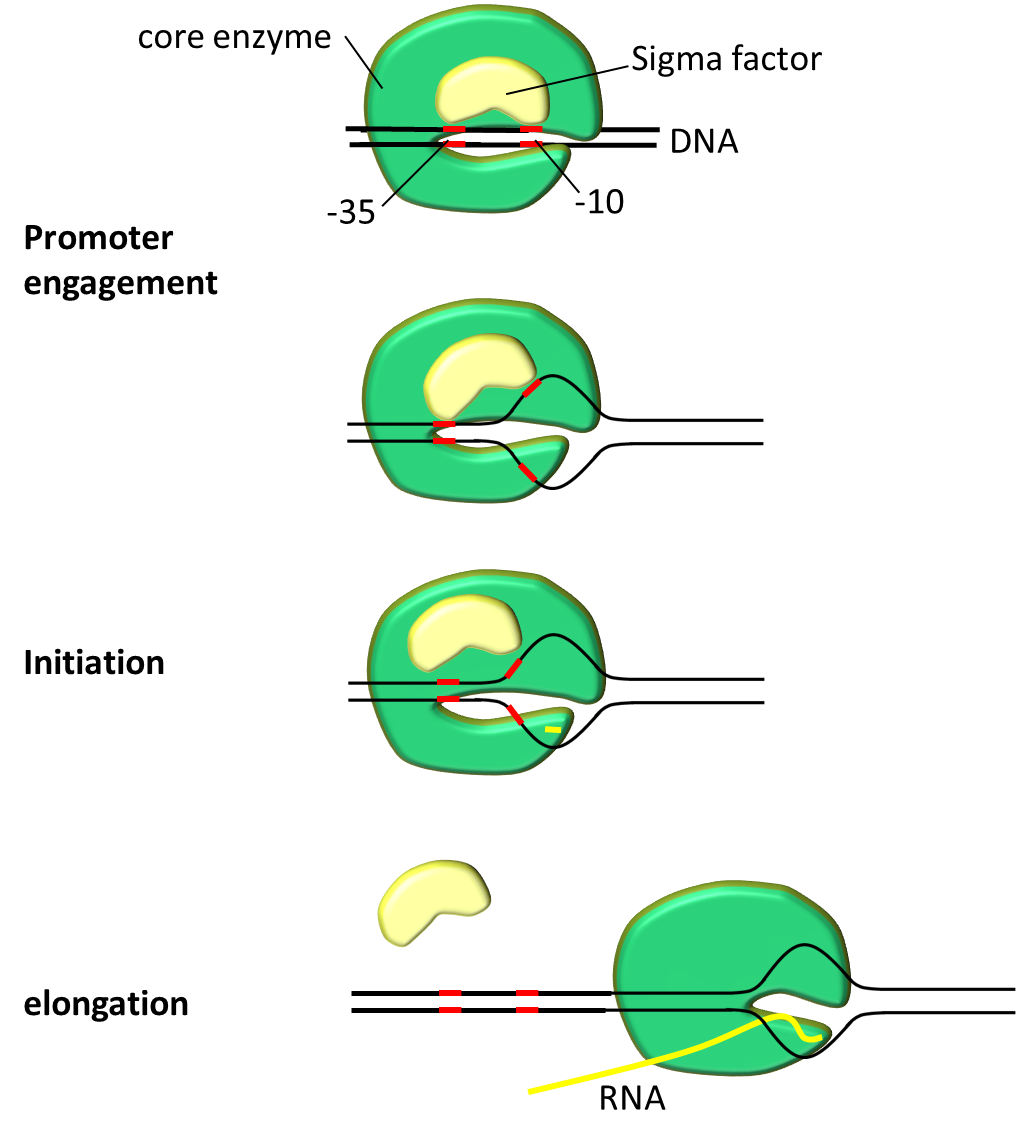Fig. 1 mRNA transcription starts with promoter engagement, continues to initiation, elongation, and then it comes to termination (omitted in the figure).

First transcription complex must be formed. Transcription complex polymerizes mRNA in 2 steps. Initiation step starts polymerization followed by elongation step. Promoter serves crucial role on engagement and initiation. After closed complex formation DNA double helix pulled apart to form transcription bubble. During this closed complex changes into open complex. This marks the beginning of mRNA polymerization. Transcription bubble exposes deoxyribonucleotides to form new hydrogen bonds with ribonucleotides. In short DNA serves as template to make mRNA.

## Transcription Factors related to Promtoer

RNA complex consist of 5 core enzymes and a sigma factor. Sigma factor plays crucial role in promoter recognition. It recognizes and binds to promoter region on DNA sequence and helps to assemble the core enzyme and start transcription. Σ factor has several analogs, E. coli which is widely used bacteria by iGEMers is using sigma;70 for house-keeping gene expression at exponential growth. Bacterial promoter can be roughly divided into three regions; -10 region, spacer and -35 region. Bases in promoter are numbered in descending order from transcription start base which is defined as +1.

-10 region
The -10 region is structurally very important because it is initiates promoter melting in RNAP-promoter complex. This is essential to form open complex. Promoter consensus sequence is TATAAT at -12 to -7 position.
Spacer
Spacer is thought to increase flexibility of sigma factor binding requirements.
-35 region
-35 region is second in importance to -10. It does not energetically contribute to promoter melting. There reports on promoters without -35 region. In those case TG motif at about -16 is thought as alternative. -35 consensus sequence is TTGACA at from -36 to -31.

Promoters function to bind RNAP is a reason it is genetically well preserved. Most frequently conserved residues in the sequence make a "consensus sequence". In 1983, -35 and -10 consensus was showed to be TTGACA and TATAAT respectively [Fig 2]. Horizontal axis of the figures represents the position upstream of translation ignition point. Letter at the top of the figure signifies more than over 39% occurrence of that letter at that position. Larger occurrence over 54% is represented as upper case letter. Consensus sequence published by Marjan De Mey et al. (2007) shows that -10 and -35 region is highly preserved [Fig 3]. There other less preserved regions. The tetramer (TRTG) upstream from -10 region is called TG motif. Upstream of -35 region is UP element and downstream of -10 region is discriminator region. These sequences are thought to bind core enzymes. So these sequences are also well conserved. Each sequence is important to control promoter strength.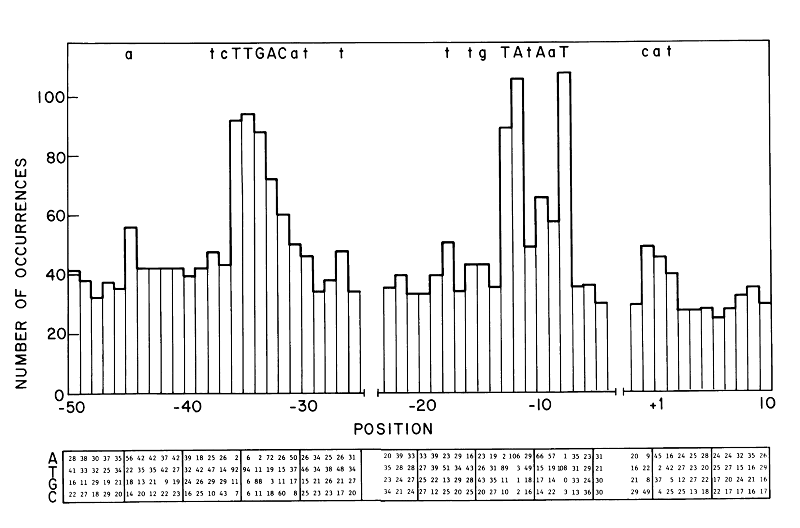Fig. 2 Consensus sequence shown in review article in 1983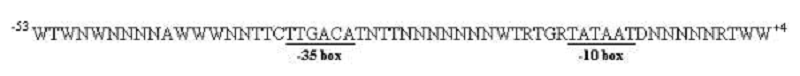Fig. 3 Consensus sequence prepared in 2007 

So we went and designed "consensus promoter". It should have strongest binding energy to RNAP. By adding mutations to -35 we sought to construct promoters with various binding energies. There are three reasons why we used -35 region.

First, -35 region is just supporting binding with σ factor. It has less vital role compared to -10 region, which energetically contributes to formation of open complex. Having this in mind we changed -35 region to easily change promoter binding strength without severe errors in promoter function.

Second, RNAP and promoter binding orchestrated by σ factor binding. Complex formation is thought to be rate-limited step. We thought that -35 region performs a simpler function. For this reason, mutations at -35 region can be thought as more structurally transparent.

Recently published research reported the making of promoter family by randomizing both -35 and -10 regions, changing spacer length. However it would be too much of the task for us to make some many changes. By changing hexamer sequence of -35 region there are 4096 variation. This number is a lot smaller compared to mutating every promoter position. So we can get result with a smaller library size.

With these 3 reasons we went on to construct our promoter family.

## Theoretic Prediction of Promoter Strength Distribution

The study by Brewster et al.  made it possible to theoretically predict the transcription efficiency using the promoter sequence, at least to a certain extent. To predict it, we need to follow these 3 steps.

1. Calculate the binding energy of promoter and sigma factor using the sequence
2. Convert the binding energy to the probability that RNAP binds promoter
3. Convert the binding probability to the transcription efficiency

Using this theory, we tried to find the strength distribution of 4096 promoters, which were artificially created by random mutation.

As the first step, we must find the binding energy of each promoter. As we mutated only -35 region, we only use this region for calculations. The binding energy is the energy needed for two bodies to bind. This is formulated below.

$\varepsilon_{\mathrm{bind}} = \Delta G = G_{\mathrm{bound} } - G_{\mathrm{unbound}}$

Provided that G stands for Gibbs free energy. This means that the lower is the binding energy, the higher is the binding strength. We referred the data in Kenney et al.  to calculate each binding energy.

The distribution of computed 4096 promoters' binding energies is shown below. The horizontal axis stands for $\varepsilon_{-35}$: the binding energy of -35 region and RNAP (at $0.05k_{B}T$ intervals) and the vertical axis sample number.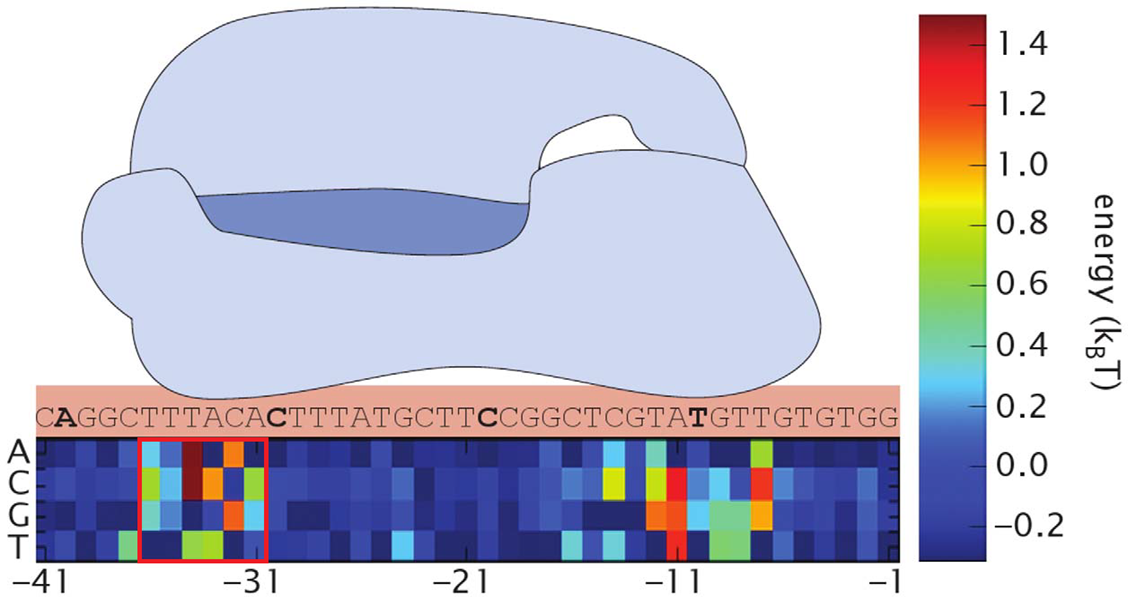M-Fig. 1 Visualized data. A portion enclosed with red square is randomized -35 region.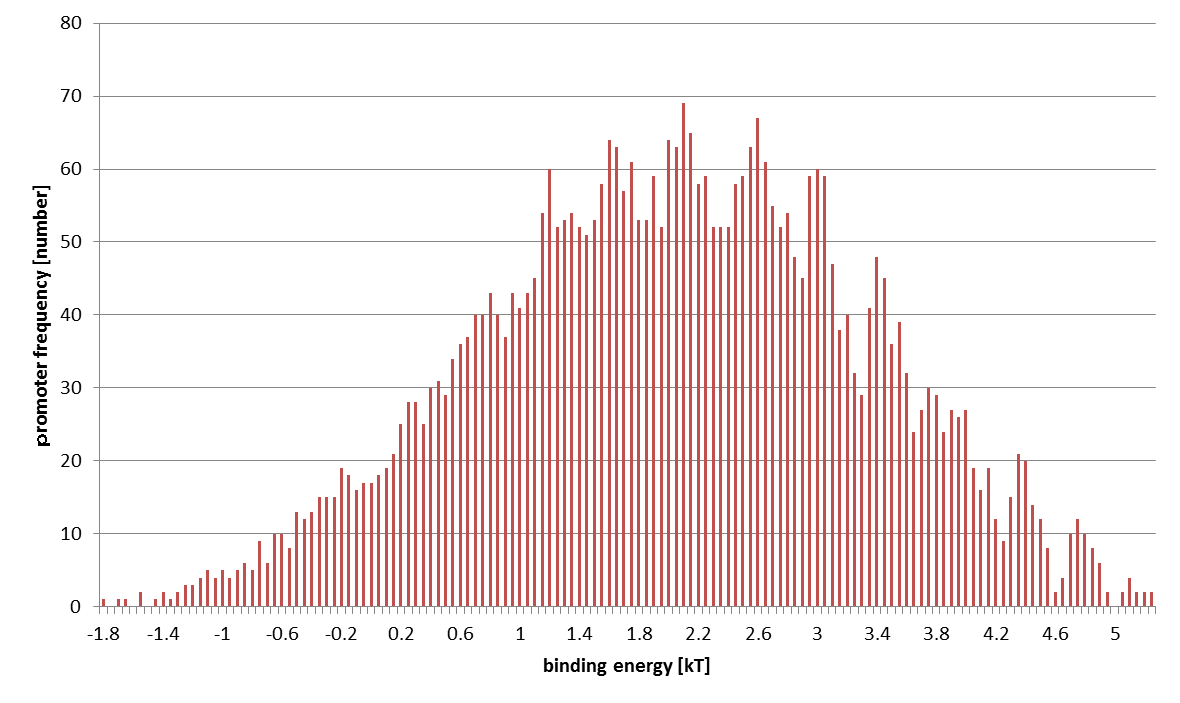M-Fig. 2 The result is an approximate normal distribution.

Next, we found RNAP's binding probability using this binding energy. To simplify the calculation, we assumed the following.

• The environment is a closed system
• P RNAPs bind somewhere on DNA
• There are $N_{\mathrm{NS}}$ non-specific binding sites and one specific binding site (=promoter) on DNA
• Define $\varepsilon_{\mathrm{NS}}$ as binding energy of RNAP and non-specific binding site
• Define $\varepsilon_{\mathrm{S}}$ as binding energy of RNAP and promoter

According to statistical mechanics, there is a relation between $p_i$, the probability of state $i$ and $E_i$, the energy of this state as the following.

$p_i \propto \exp\left(-\frac{E_i}{k_{\mathrm{B}}T}\right)$

This fact gives the following calculation result.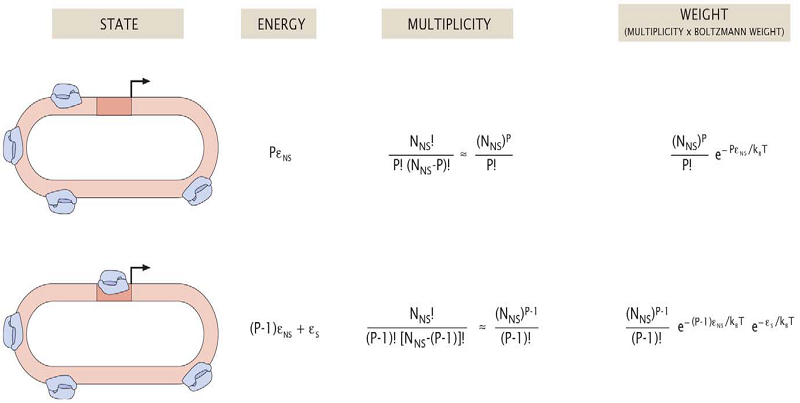M-Fig. 3 Quoted from 

Therefore, the binding probability is

\begin{align*} p&=\frac{W_{\mathrm{bound}}}{W_{\mathrm{unbound}}+W_{\mathrm{bound}}} \\[6pt] &=\frac{ \frac{P}{N_{\mathrm{NS}}} \exp\left(-\frac{\varepsilon_{\mathrm{S}} - \varepsilon_{\mathrm{NS}}}{k_{\mathrm{B}}T} \right) }{1+\frac{P}{N_{\mathrm{NS}}} \exp\left(-\frac{\varepsilon_{\mathrm{S}} - \varepsilon_{\mathrm{NS}}}{k_{\mathrm{B}}T} \right) } \\[6pt] \mathrm{suppose\ that} &\frac{P}{N_{\mathrm{NS}}} \exp\left(-\frac{\varepsilon_{\mathrm{S}} - \varepsilon_{\mathrm{NS}}}{k_{\mathrm{B}}T} \right) \ll 1 \\[6pt] &\approx \frac{P}{N_{\mathrm{NS}}} \exp\left(-\frac{\varepsilon_{\mathrm{S}} - \varepsilon_{\mathrm{NS}}}{k_{\mathrm{B}}T} \right) \\[6pt] &\propto \exp\left(-\frac{\varepsilon_{-35}}{k_{\mathrm{B}}T} \right) \end{align*}

The binding energy of -35 region is exponentially proportional to the binding probability.

The last step is to convert the binding probability to the transcription efficiency. Let us assume these suppositions.

• RNAP bound to promoter promptly initiate transcription
• There is no "traffic jam" of RNAPs on DNA (i. e., RNAP's transcription initiation is rate-limiting)

These assumptions mean that we can directly use the value of binding probability as transcription energy in an arbitrary unit. In this way, we get following conclusive result.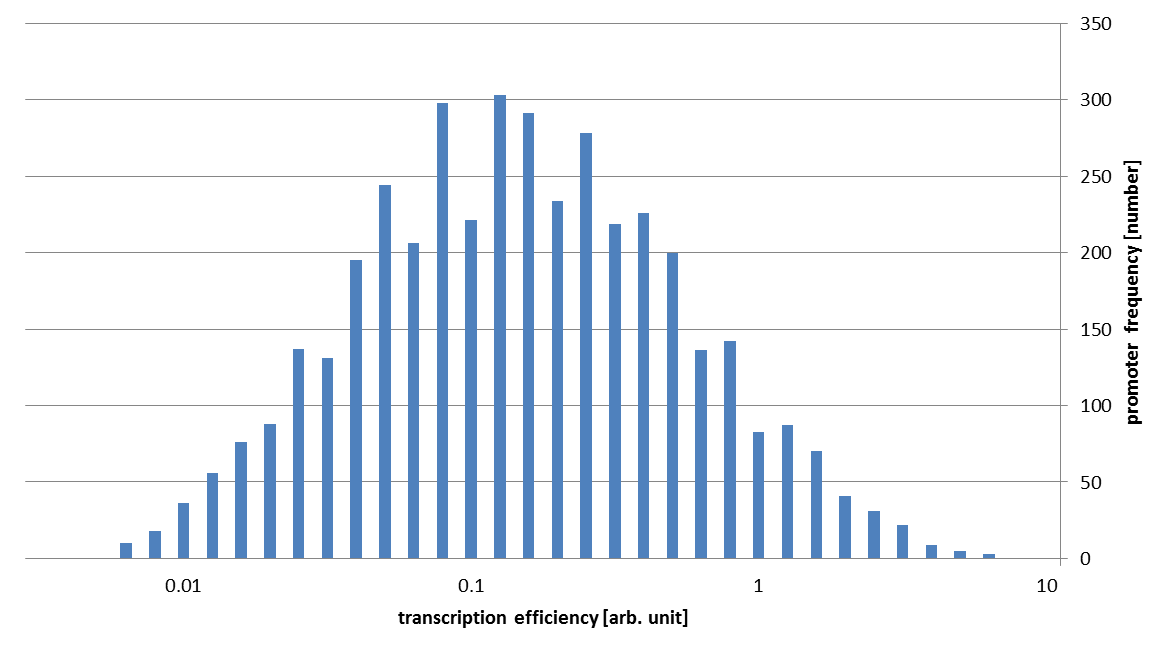M-Fig. 4 The horizontal axis stands for the transcription efficiency.

As you can see in this figure, the strengths of our promoter families vary about 1000 fold!